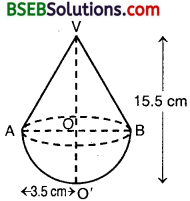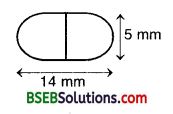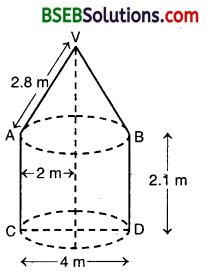# Bihar Board Class 10th Maths Solutions Chapter 13 Surface Areas and Volumes Ex 13.1

Bihar Board Class 10th Maths Solutions Chapter 13 Surface Areas and Volumes Ex 13.1 Textbook Questions and Answers.

## BSEB Bihar Board Class 10th Maths Solutions Chapter 13 Surface Areas and Volumes Ex 13.1Question 1.
2 cubes each of volume 64 cm³ are joined end to end. Find the surface area of the resulting cuboid.
Solution:
Let the length of each edge of the cube be a cm.
Then, Volume = 64 cm³ So, a³ = 64 or a = 4
When two cubes of equal volumes (i.e equal edges) are joined end to end, we get a cuboid such that its
l = Length = 4 cm + 4 cm = 8 cm
b = Breadth = 4 cm
and, h = Height = 4 cm
∴Surface area of the cuboid = 2(lb + bh + hl)
= 2(8 x 4 + 4 x 4 + 4 x 8) cm²
= 2(32 + 16 + 32) cm²
= (2 x 80) cm² = 160 cm².

Question 2.
A vessel is in the form of a hollow hemisphere mounted by a hollow cylinder. The diameter of the hemisphere is 14 cm and the total height of the vessel is 13 cm. Find the inner surface area of the vessel.
Solution:
Here, the radius r of hemi – sphere $$\frac { 14 }{ 2 }$$ = 7
the height h of cylinder = (13 – 7) cm = 6 cm.
Clearly, radius of the base of cylindrical part is also r = 7cm.Surface area of the vessel
= Curved surface area of the cylindrical part + Curved surface area of hemispherical part
= (2πh + 2π²) = 2π(h + r)
= 2 x $$\frac { 22 }{ 7 }$$ x 7 x 13 cm²
= 572 cm².Question 3.
A toy is in the form of a cone of radius 3.5 cm mounted on a hemisphere of same radius. The total height of the toy is 15.5 cm. Find of the toy.
Solution:
We have : VO’ = 15.5 cm and OA = 00′ = 3.5 cm.
Let r be the radius of the base of cone and h be the height of conical part of the toy. Then, r = OA = 3.5 cm,h = VO = VO’ – OO’
= (15.5 – 3.5) cm = 12 cm
Also, radius of the hemisphere = OA = r = 3.5 cm.
Total surface area of the toy = Curved surface area of cone + Curved surface area of hemisphere
= πrl + 2πr² = πr(l + 2 r), where l – Slant height of the cone.
Now, l = $$\sqrt{\mathrm{OA}^{2}+\mathrm{OV}^{2}}$$ = $$\sqrt{(3.5)^{2}+12^{2}}$$
= $$\sqrt{12.25+144}$$ = $$\sqrt{156.25}$$ = 12.5 cm
Therefore, surface area of the toy = πr(l + 2r)
= $$\frac { 22 }{ 7 }$$ x 3.5 x (12.5 + 2 x 3.5) cm²
= $$\frac { 22 }{ 7 }$$ x 3.5 x 19.5 cm² = 214.5 cm².

Question 4.
A cubical block of side 7 cm is surmounted by a hemisphere. What is the greatest diameter the hemisphere can have? Find the surface area of the solid.
Solution:
The greatest diameter that a hemisphere can have = 7 cm.
Surface area of the solid after surmounting hemisphere = 6l² – TCR² + 2πR², where l = side of cubical block and R = radius of hemisphereQuestion 5.
A hemispherical depression is cut out from one face of a cubical wooden block such that the diameter l of the hemisphere is equal to the edge of the cube. Determine the surface area of the remaining solid.
Solution:
Edge of the cube = l
Diameter of the hemsiphere = l
∴ Radius of the hemisphere = $$\frac { l }{ 2 }$$
∴ Area of the remaining solid after cutting out the hemispherical depression
= 6l² – π($$\frac { l }{ 2 }$$)² + 2π($$\frac { l }{ 2 }$$)
= 6l² + π($$\frac { l }{ 2 }$$)²
= 6l² + π x $$\frac{l^{2}}{4}$$
= $$\frac{l^{2}}{4}$$(24 + π)Question 6.
A medicine capsule is in the shape of a cylinder with two hemispheres stuck to each of its ends (See figure). The length of the entire capsule is 14 mm and the diameter of the capsule is 5 mm.Find its surface area.
Solution:
Let r mm be the radius and h mm be the height of the cylinder. Then,r = $$\frac { 5 }{ 2 }$$ mm = 2.5 mm
and, h = (14 – 2 x $$\frac { 5 }{ 2 }$$) mm
= (14 – 5) mm = 9 mm
Also, the radius of hemisphere r = $$\frac { 5 }{ 2 }$$ cm
Now, surface area of the capsule = Curved surface area of cylinder + Surface area of two hemispheres
= (2πrh + 2 x 2πr²)
= 2πr(h + 2 r)
= 2 x $$\frac { 22 }{ 7 }$$ x $$\frac { 5 }{ 2 }$$ x (9 + 2 x $$\frac { 5 }{ 2 }$$) mm²
= $$\frac { 22 }{ 7 }$$ x 5 x 14 mm² = 220 mm²

Question 7.
A tent is in the shape of a cylinder surmounted by a conical top. If the height and diameter of the cylindrical part are 2.1 m and 4 m respectively, and the slant height of the top is 2.8 m, find the area of the canvas used for making the tent. Also, find the cost of the canvas of the tent at the rate of Rs 500 per m². (Note that the base of the tent will not be covered with canvas.)
Solution:
We have : Total canvas used = Curved surface area of cylinder + Curved surface area of cone= (2πrh + πrl)
= πr(2h + l)
= $$\frac { 22 }{ 7 }$$ x 2 x ( 2 x 2.1 + 2.8) m²
= $$\frac { 22 }{ 7 }$$ x 2 x 7 m²
= 44 m²
Now, cost of 1 m² canvas for the tent = Rs 500
So, cost of 44 m² canvas for the tent = Rs 44 x 500 = Rs 22000Question 8.
From a solid cylinder whose height is 2.4 cm and diameter 1.4 cm, a conical cavity of the same height and same diameter is hollowed out. Find the total surface area of the remaining solid to the nearest cm².
Solution:
Radius of the cylinder = $$\frac { 14 }{ 2 }$$ cm – 0.7 cm
Height of the cylinder = 2.4 cm
Radius of the cone = 0.7 cm
Height of the cone = 2.4 cm
Slant height of the coneSurface area of the remaining solid = Curved surface area of cylinder + Curved surface area of the cone + Area of upper circular base of cylinder
= 2πrh + πrl + πr2 = πr(2h + l + r)
= $$\frac { 22 }{ 7 }$$ x 0.7 x (2 x 2.4 + 2.5 + 0.7) cm²
= 22 x 0.1 x (4.8 + 2.5 + 0.7) cm²
= 2.2 x 8.0 cm²
= 17.6 cm²
= 18 cm²Question 9.
A wooden article was made by scooping out a hemisphere from each end of a solid cylinder, as shown in figure. If the height of the cylinder is 10 cm, and its base is of radius 3.5 cm, find the total surface area of the article.Solution:
Surface area of the article when it is ready = Curved surface area of cylinder + 2 x Curved surface area of hemisphere= 2 πrh + 2 x 2πr²
= 2πr(h + 2r), where r = 3.5 cm and h = 10 cm
= 2 x $$\frac { 22 }{ 7 }$$ x 3.5 x (10 + 2 x 3.5) cm²
= 2 x $$\frac { 22 }{ 7 }$$ x 3.5 x 17 cm² = 374 cm².